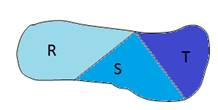Chapter 8.1, Problem 44E### Elementary Geometry for College St...

6th Edition
Daniel C. Alexander + 1 other
ISBN: 9781285195698

#### Solutions

Chapter
Section### Elementary Geometry for College St...

6th Edition
Daniel C. Alexander + 1 other
ISBN: 9781285195698
Textbook Problem
1 views

# Given region R ∪ S ∪ T , explain why A R ∪ S ∪ T > A R + A S + A T .To determine

To explain:

ARST=AR+AS+AT

If region RST is given byExplanation

Given,

From the figure, region R, S, and T are mutually exclusive and exhaustive.

(i.e.) RST

Where RST=R+S+T

Area of the region R, S, and T is AR,AS, and AT.

Thus, the area of the figure is ARST

(i

### Still sussing out bartleby?

Check out a sample textbook solution.

See a sample solution

#### The Solution to Your Study Problems

Bartleby provides explanations to thousands of textbook problems written by our experts, many with advanced degrees!

Get Started